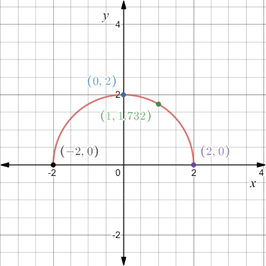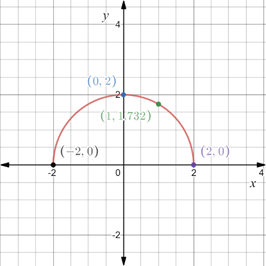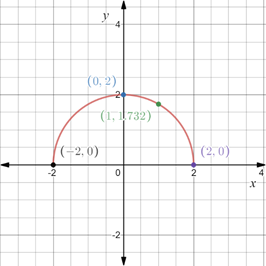# The x- and y- intercepts for the equation y = 4 − x 2 and test the symmetry of the equation. Also construct the table to sketch the graph of the equation.### Precalculus: Mathematics for Calcu...

6th Edition
Stewart + 5 others
Publisher: Cengage Learning
ISBN: 9780840068071### Precalculus: Mathematics for Calcu...

6th Edition
Stewart + 5 others
Publisher: Cengage Learning
ISBN: 9780840068071

#### Solutions

Chapter 1.8, Problem 69E
To determine

## To calculate: The x- and y- intercepts for the equation y=4−x2 and test the symmetry of the equation. Also construct the table to sketch the graph of the equation.

Expert Solution

The x-intercepts are ±2 and y-intercept is 2 . The equation is symmetric about y-axis.Graph of the equation y=4x2 is provided below,### Explanation of Solution

Given information:

The equation y=4x2 .

Formula used:

The function is symmetric about the x-axis, when y is replaced by y , the equation remains unchanged.

The function is symmetric about the y-axis, when x is replaced by x , the equation remains unchanged.

The function is symmetric with respect to origin, when y is replaced by y and x is replaced by x , the equation remains unchanged.

The x-intercepts are the points on x-axis where the graph of the equation intersects the x-axis.

The y-intercepts are the points on y-axis where the graph of the equation intersects the y-axis.

Calculation:

It is provided that the equation is y=4x2 . Construct a table to evaluate the value of y for different values of x.

Substitute the point x=2 in the equation y=4x2 ,

y=4(2)2y=44y=0

Substitute the point x=0 in the equation y=4x2 ,

y=402y=4y=2

Substitute the point x=1 in the equation y=4x2 ,

y=4(1)2y=41y=3

Substitute the point x=2 in the equation y=4x2 ,

y=4(2)2y=44y=0

Construct a table with the values obtained above,

xy(x,y)20(2,0)02(0,2)13(1,3)20(2,0)

In the coordinate plane plot the points obtained above and connect them through a line.

The graph of the equation is provided below y=4x2 .Recall that the x-intercepts are the points on x-axis where the graph of the equation intersects the x-axis.

Substitute y=0 in the equation y=4x2 ,

0=4x24x2=0x2=4x=±2

Therefore, x-intercepts are ±2 .

Recall that the y-intercepts are the points on x-axis where the graph of the equation intersects the y-axis.

Substitute x=0 in the equation y=4x2 ,

y=402y=4y=2

Therefore, y-intercept is 2 .

Recall that the function is symmetric about the x-axis, when y is replaced by y , the equation remains unchanged.

Replace y by y in the equation y=4x2 ,

y=4x2

The equation is changed. Therefore, the equation y=4x2 is not symmetricabout the x-axis.

Recall that the function is symmetric about the y-axis, when x is replaced by x , the equation remains unchanged.

Replace x by x in the equation y=4x2 ,

y=4(x)2y=4x2

The equation is unchanged. Therefore, the equation y=4x2 is symmetricabout the y-axis.

Recall that the function is symmetric with respect to origin, when y is replaced by y and x is replaced by x , the equation remains unchanged.

Replace x by x and y by y in the equation y=4x2 ,

y=4(x)2y=4x2

The equation is changed. Therefore, the equation y=4x2 is not symmetricabout the origin.

Thus, the x-intercepts are ±2 and y-intercept is 2 . The equation is symmetric about y-axis.Graph of the equation y=4x2 is provided below,### Have a homework question?

Subscribe to bartleby learn! Ask subject matter experts 30 homework questions each month. Plus, you’ll have access to millions of step-by-step textbook answers!